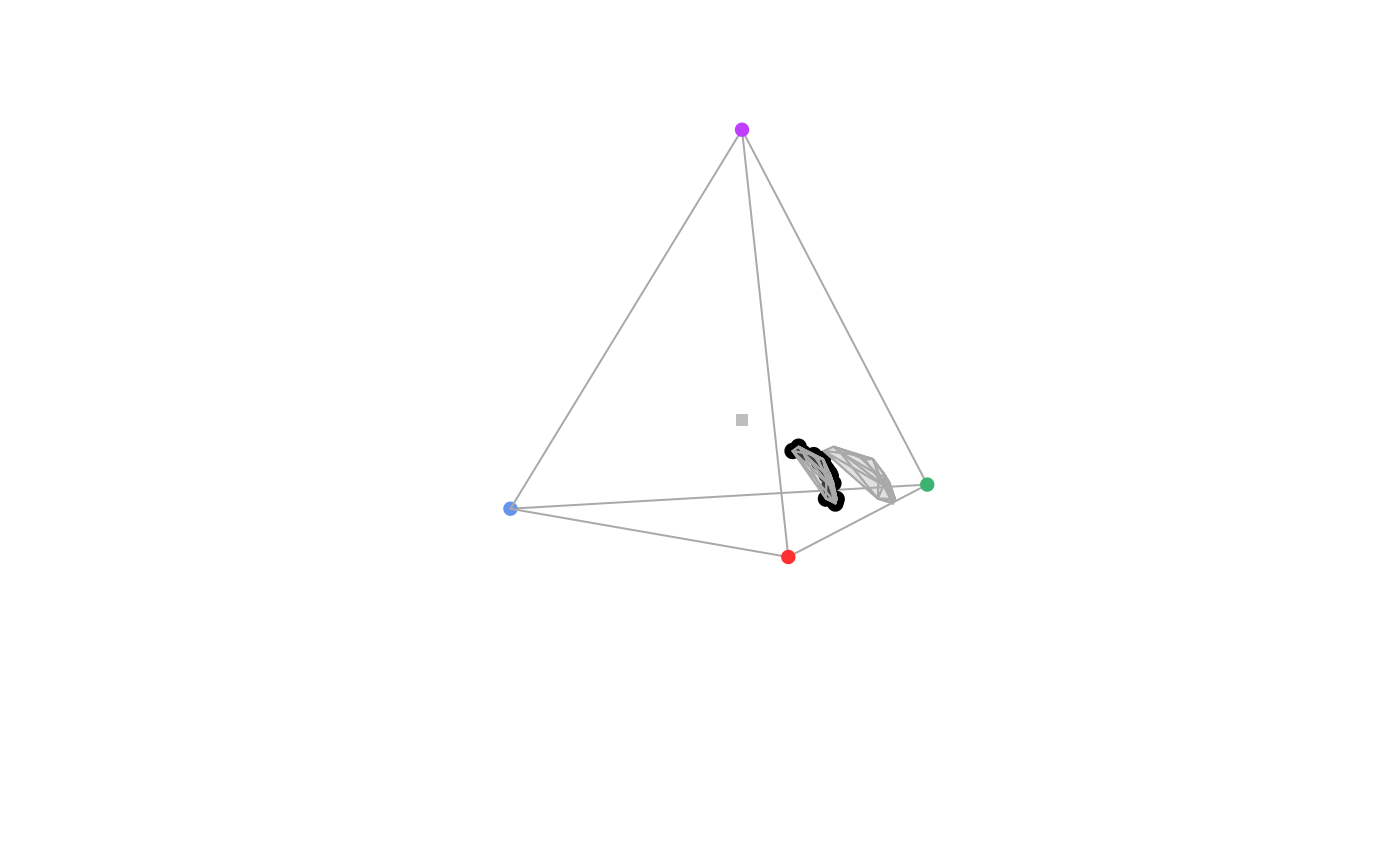Produces a 3D colour volume in tetrahedral colour space when plotting a non-interactive tetrahedral plot.

vol(
tcsdata,
type = c("convex", "alpha"),
avalue = "auto",
alpha = 0.2,
grid = TRUE,
fill = TRUE,
new = FALSE,
...
)

## Arguments

tcsdata (required) a data frame, possibly a result from the colspace() or tcspace() function, containing values for the 'x', 'y' and 'z' coordinates as columns (labeled as such). if "convex", the colour volume is plotted using a convex hull and if "alpha", it is plotted using alphashapes. if type = "alpha", which alpha parameter value should be used to compute the alphashape. avalue = "auto" (default) finds and use the α* value as defined in Gruson (2020). transparency of volume (if fill = TRUE). logical. if TRUE (default), draws the polygon outline defined by the points. logical. if TRUE (default), fills the volume defined by the points. logical. Should a new plot be started or draw over an open plot? (defaults to FALSE) additional graphical options. See polygon() and tetraplot().

## Value

vol() creates a 3D colour volume within a static tetrahedral plot.

Stoddard, M. C., & Prum, R. O. (2008). Evolution of avian plumage color in a tetrahedral color space: A phylogenetic analysis of new world buntings. The American Naturalist, 171(6), 755-776.

Stoddard, M. C., & Stevens, M. (2011). Avian vision and the evolution of egg color mimicry in the common cuckoo. Evolution, 65(7), 2004-2013.

Maia, R., White, T. E., (2018) Comparing colors using visual models. Behavioral Ecology, ary017 doi: 10.1093/beheco/ary017

Gruson H. 2020. Estimation of colour volumes as concave hypervolumes using α-shapes. Methods in Ecology and Evolution, early view doi: 10.1111/2041-210X.13398

## Author

Rafael Maia rm72@zips.uakron.edu

Hugo Gruson

## Examples


# For plotting
data(sicalis)
vis.sicalis <- vismodel(sicalis, visual = "avg.uv")
tcs.sicalis <- colspace(vis.sicalis, space = "tcs")
plot(tcs.sicalis)

# Convex hull
vol(tcs.sicalis, type = "convex")

# Alpha-shape
if (require("alphashape3d")) {
vol(tcs.sicalis, type = "alpha", avalue = 1)
}
#> Loading required package: alphashape3d#> Loading required package: geometry#> Loading required package: rgl